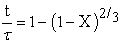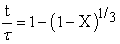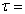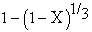﻿ Kinetics of Simultaneous Dissolution of Zinc and Manganese from Electrolyte Paste of Spent Zinc-Carbon Dry Cell Battery in Sulfuric Acid Solution

### Kinetics of Simultaneous Dissolution of Zinc and Manganese from Electrolyte Paste of Spent Zinc-Carb...

Majharul Haque Khan, ASW KurnyOPEN ACCESSPEER-REVIEWED

## Kinetics of Simultaneous Dissolution of Zinc and Manganese from Electrolyte Paste of Spent Zinc-Carbon Dry Cell Battery in Sulfuric Acid Solution

Majharul Haque Khan1, ASW Kurny1,1Department of Materials and Metallurgical Engineering Bangladesh University of Engineering and Technology, Dhaka, Bangladesh

### Abstract

Manganese and zinc contained in the electrolyte paste of spent zinc-carbon dry cell batteries were leached in sulfuric acid in presence of hydrogen peroxide as a reducing agent. Kinetic parameters were established from the time versus extent of dissolution curves using temperature as variable and keeping the other parameters fixed. Three reactions models (i) Liquid film diffusion controlled, (ii) Diffusion controlled through the product layer and (iii) Chemical reaction controlled were considered for the selection of the appropriate reaction model for the dissolution of manganese and zinc. The kinetic data of leaching for both manganese and zinc were found to follow the chemical reaction controlled process, i..e.,where, τ = time for complete disappearance of particles and X = fraction of reacted particles. Activation energy of manganese and zinc were found to be 46.27 KJ/mol and 52.39 KJ/mol respectively; which again justified the appropriateness of the model. At a leaching temperature of 60°C, the extent of dissolution, under the conditions investigated, reached up to 81% for manganese within 21 minutes of leaching and 75% for zinc within 30 minutes of leaching.

### At a glance: Figures

1234
Prev Next

• Khan, Majharul Haque, and ASW Kurny. "Kinetics of Simultaneous Dissolution of Zinc and Manganese from Electrolyte Paste of Spent Zinc-Carbon Dry Cell Battery in Sulfuric Acid Solution." Materials Science and Metallurgy Engineering 2.1 (2014): 1-4.
• Khan, M. H. , & Kurny, A. (2014). Kinetics of Simultaneous Dissolution of Zinc and Manganese from Electrolyte Paste of Spent Zinc-Carbon Dry Cell Battery in Sulfuric Acid Solution. Materials Science and Metallurgy Engineering, 2(1), 1-4.
• Khan, Majharul Haque, and ASW Kurny. "Kinetics of Simultaneous Dissolution of Zinc and Manganese from Electrolyte Paste of Spent Zinc-Carbon Dry Cell Battery in Sulfuric Acid Solution." Materials Science and Metallurgy Engineering 2, no. 1 (2014): 1-4.

 Import into BibTeX Import into EndNote Import into RefMan Import into RefWorks

### 1. Introduction

Zinc-carbon and alkaline dry cell batteries are the most used type batteries and are widely used in toys, radios, recorders, watches, remote controls, cameras, torches etc. These batteries are discarded at the end of useful life. The amount of waste, which is generated everyday by these batteries in the world, is significant. Zinc-carbon dry cell batteries are therefore, considered to be a valuable secondary source of metals like zinc and manganese. Consequently, there has been an increasing interest in developing processes for the recovery of the metal values from the spent zinc-carbon dry cell batteries. Both pyrometallurgical and hydrometallurgical processes have been employed for treating such secondary raw materials. Hydrometallurgical routes are considered to be more environment friendly.

Leaching is the first step in hydrometallurgy to recover value metals and leaching kinetics is important from the economic point of view. Acid leaching is generally preferred, in hydrometallurgical route of separation of the value metals from spent zinc-carbon type batteries. Sulfuric acid leaching in presence of a reducing agent has been tried by many researchers for efficient dissolution of manganese and zinc from the electrolyte paste of such batteries [1, 2]. Investigations on the kinetics parameters have been of special interest to many researchers to determine the rate of reaction and rate controlling steps [3, 4].

The progressive conversion model and the shrinking core model are the two simple models generally considered for the non-catalytic reaction of particles with the surrounding fluid. In the progressive conversion model, reaction is assumed to occur throughout the particles at different rates at different locations, which is unrealistic in maximum leaching experiments . Hence, shrinking core model is preferred for solid spherical particle reaction kinetics. Liquid film diffusion controlled, diffusion controlled process through the product layer and the chemical reaction controlled processes are the three models generally considered for the preliminary selection of kinetic models Figure 3. Generally a kinetic model is matched with the results of leaching experiments, and taking data from this model, activation energy is determined .

In the present study, both manganese and zinc contained in the electrolyte paste in the spent zinc-carbon dry cell batteries have been simultaneously leached in sulfuric acid media in the presence of hydrogen peroxide as a reducing agent. The reaction mechanism has been identified and the activation energy for dissolution of both manganese and zinc has been estimated.

### 2. Experimental

Electrolyte paste of the spent zinc-carbon dry cell batteries were separated manually. The paste collected from different types of zinc carbon dry cell batteries were mixed together. The mixed paste was dried in an oven at 110°C to a constant weight and sieving was done to get a definite particle size for subsequent leaching and kinetic studies. Particles in the range of -100 to +140 mesh size (0.104 to 0.152 mm) were used in the leaching experiments. The sample of paste selected for study contained 22 per cent zinc and 60 percent manganese.

Leaching was conducted in a 500-ml three necked round bottom flask fitted with a mechanical stirrer, temperature sensor and refluxing condenser. The solution was heated by a mantle heater. Initially leaching was performed to identify the optimum process parameters. Subsequent leaching was performed under the following optimum conditions: Concentration of H2SO4 = 2.5M, Concentration of H2O2 = 6%, stirring speed = 400 rpm and solid/liquid ratio = 1:5. The rate controlling steps for kinetics study were estimated from the effect of temperature in the range 40 – 60oC, on the extent of dissolution of manganese and zinc. Potassium per-iodate (KIO4) was used as a complexing agent for analysis of manganese by a Shimadzu UV-vis spectrophotometer. Zinc was analyzed in a SensAA atomic absorption spectroscopic machine. Activation energy was determined from Arrhenius equation for manganese and zinc.

### 3. Results and Discussion

3.1. Effects of Reaction Temperature

Three temperatures (40°C, 50°C and 60°C) were considered for the study of kinetics of leaching of manganese and zinc in sulfuric acid media, the other leaching variables were kept constant. Hydrogen per oxide was added to the solution as a reducing agent. Leaching behavior of manganese and zinc at leaching temperatures in the range 40°C to 60°C is shown in Figure 1 and Figure 2.

Above 60°C, hydrogen peroxide dissociates and extraction efficiency of both manganese and zinc drastically drops to a lower value . The initial rate of dissolution of both the elements, manganese and zinc, was very fast, which decreased slowly towards the end of leaching. Dissolution of manganese reached up to 81% within 21 minutes of the beginning of leaching, while the dissolution of zinc reached 75% within 30 minutes of leaching at 60°C.

3.2. Kinetic Models

The particles were assumed to be perfectly homogeneous spherical solid phases and shrinking core model was selected to analyze the leaching rate of manganese in solution . Three previously established models, i.e., liquid film diffusion controlled process, diffusion controlled process through the product layer and chemical reaction controlled process were considered for initial selection of the reaction mechanism. The equations of these models can be expressed as follows :

1. Liquid film diffusion controlled(1)

2. Diffusion controlled through the product layer(2)

3. Chemical reaction controlled(3)

Where,Time for complete disappearance of particles

And X = Fraction of reacted particles

3.2.1. Liquid Film Diffusion Controlled Process

Time for different fraction of reacted particles of manganese and zinc at 40°C, 50°C and 60°C were calculated from Figure 1 and Figure 2. Data was then plotted according to the Equation 1. Correlation coefficient values of the plotted lines are also shown in Figure 3 and Figure 4. However, the correlation is not high enough for all the temperatures under consideration to suggest that the liquid film diffusion controlled process is the appropriate model for leaching of manganese and zinc. At lower temperature, correlation coefficient values were much higher for both manganese and zinc. But random coefficient values were observed at higher temperatures.

3.2.2. Diffusion Controlled Process Through the Product Layer

According to this model, the core of particle diminishes while the overall particle size remains constant. Also, the reaction is fast at the initial level due to easy diffusion of the reactant inside the particle and then diminishes slowly, as the product layer in the later stages controls the diffusion process. In order to test the possibility of diffusion through a solid reaction product, the right hand side of the Equation 2 was plotted against time for manganese (Figure 5) and for zinc (Figure 6).

The correlation factors for the three temperatures under consideration were very low and the curves did not pass through the zero point. The diffusion through the solid reaction product could not therefore, be the rate determining step during leaching of manganese and zinc in sulfuric acid solutions.

3.2.3. Chemical Reaction Controlled process

Chemical reaction controlled process assumes that the reaction in the spherical particle, in leaching solution occurs homogeneously in all direction. The plot ofversus time is shown in Figure 7 and Figure 8 for leaching of manganese and for zinc respectively. The data yielded a very high correlation coefficient for all the temperatures under investigation for both manganese and zinc. Moreover, the plotted lines at different temperatures pass through the exact zero point. Therefore, the data could be correlated to a chemical reaction controlled process.

3.3. Activation Energy Determination

Reaction rate constants (K) at various temperatures were obtained from the slope of the linear plots in Figure 7 and Figure 8 for manganese and zinc respectively. Then the values of reaction rate constants (K) were plotted according to the Arrhenius type equation. The plot of ln K vs. 1/T (K) was straight line for both manganese and zinc. This is shown in Figure 9 and Figure 10. The apparent activation energy, as estimated from the Arrhenius type plot, was 46.27 KJ/mol for manganese and 52.39 KJ/mol for zinc.

Figure 10. Arrhenius type plot for zinc leaching kinetic data of Figure 8

The typical activation energy for a diffusion controlled process is generally less than 40 kJ/Kg and, the activation energy is generally more than 40 kJ/Kg for a chemically controlled process . However, it has been previously stated that activation energy alone does not precisely determine a fixed reaction kinetics model but is only a guide to predict it . As the activation energy for manganese is 46.27 KJ/mol and for zinc is 52.39 KJ/mol, it may be concluded that the chemical reaction controlled process, as selected should be the appropriate model for dissolution of both manganese and zinc in sulfuric acid solutions containing hydrogen per oxide.

### 4. Conclusion

Dissolution of manganese and zinc contained in the electrolyte paste of spent zinc-carbon dry cell batteries in sulfuric acid solutions containing hydrogen peroxide as a reducing agent was investigated. The reaction mechanism was identified and the activation energy was determined. The following conclusions can be drawn from the study:

1. An increase in temperature up to 60°C increased the rate of leaching of both manganese and zinc.

2. The dissolution of both manganese and zinc in sulfuric acid solution was found to be chemical reaction controlled.

3. Activation energies of manganese and zinc were found to be 46.27 KJ/mol and 52.39 KJ/mol respectively, which confirmed that the dissolution of manganese and zinc was chemical reaction controlled.

### Acknowledgements

The authors are grateful to Dr. Abdul Gafur of Bangladesh Council of Scientific and Industrial Research (BCSIR), Dhaka for his cooperation during this study. The authors are also grateful to the authorities of Bangladesh University of Engineering and Technology (BUET) for providing funds for this work.

### References

  Ferella, F., Michelis, I. De, Beolchini, F., Innocenzi, V., Veglio F., “Extraction of zinc and manganese from alkaline and zinc-carbon batteries by citric-sulfuric acid solution”, Int. J. of Chemical Engineering, Vol. 2010, Article ID 659434.In article  Zhang, W. and Cheng, C.Y., “Manganese metallurgy review. Part II: Manganese separation and recovery from solution”, Hydrometallurgy, 2007, 89, 160.In article CrossRef  Avraamides, J., Senanayake, G., Clegg, R., “Sulfur dioxide leaching of spent zinc-carbon-battery scrap”, J. of Power Source, 2006, 159, 1488.In article CrossRef  Baba, A. A., Adekola, A. F., Bale, R. B., “Development of a combined pyro- and hydro-metallurgical route to treat spent zinc-carbon batteries”, J. of Hazardous Materials, 2009, 171, 838.In article CrossRef PubMed  Levenspiel, O., “Chemical reaction engineering”, 3rd edition, John Wiley & Sons. : New York, 1999.In article  Ige, J., Akanni, M.S., Morakinyo, M. K., Owoyomi, O., “A kinetic study of the leaching of iron and manganese from Nigerian tantalite-columbite ore”, J. of Applied Science, 2005, 5(3), 496-502.In article CrossRef  Adebayo, A.O., Ipinmoroti, K. O., Ajayi, O. O., “Leaching of sphalerite with hydrogen peroxide and nitric acid solutions”, J. of Minerals and Materials Characterization & Engineering, 2006, 5(2), 167.In article  Espiari, S., Rashchi, F., Sadrnezhaad, S. K., “Hydrometallurgical treatment of tailings with high zinc content”, Hydrometallurgy, 2006, 82, 54.In article CrossRef  Ray, H. S., “Kinetics of Metallurgical Reactions”, Oxford & IBH Publishing: India, 1993.In article  Shin, S. M., Sohn, J. S., “The behavior of valuable metals from spent zinc-carbon batteries in sulfuric acid solution”, J. Korea Society of Waste Management, 2005, 10 (1), 25.In article  Espiari, S., Rashchi, F., Sadrnezhaad, S. K., “Hydrometallurgical treatment of tailings with high zinc content”, Hydrometallurgy, 2006, 82, 54.In article CrossRef  Abdel-Aal, E.A., “Kinetics of sulfuric acid leaching of low grade zinc silicate ore”, Hydrometallurgy, 2000, 55, 247.In article CrossRef